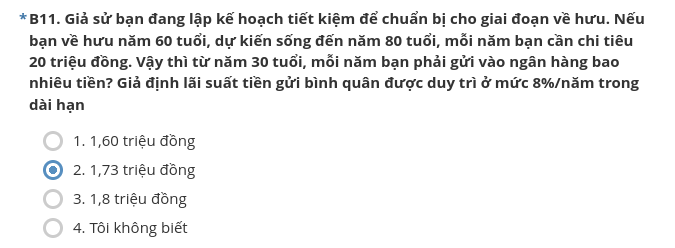# Saving tips?... Maybe?

Tags: math

I recently received a survey from VASS about student’s awareness of personal finance. I found an interesting quiz that at first make no sense until I took my pen and actually start work on it.

### The problem

Assuming that you are setting a saving plans for your retirement at 60. If you continues to live until 80 and spends 20 million VND each year, how much would you save in a bank each year, starts from your 30 and given that the rate is 8% and there will be no inflation.### Initial thoughts on the quiz

At first, I give it a vague guess by removing the base rate. As you taking total of 400 millions over the last 20 years, you would have to deposit 13.33 millions per year to make up for that savings. However, the only four options I received was:

• 1.60 mil/year
• 1.73 mil/year
• 1.80 mil/year
• Don’t know

Erm… what? How come you only saves under 2 mil per year for 30 years and having access to that large savings? Is the rate of 8% gives around 7 times (1.8 compared to 13.3) your money? Yes, it actually does, and the fun fact is that you have excess money at 80 if you opt to deposit 1.8 millions per year. Sounds ridiculous? That’s the story of interest rates.

Notes: The problem is nowhere near realistic situation since no inflation is involved in calculation.

### Let’s start (seriously) do the math, shall we?

Let $$s$$ be our annually saving amount. We start at the end of age 30 and with the interest rate of 8% per year. We could compute each year’s money till we retired

Age Saving
$$30$$ $$s$$
$$31$$ $$1.08s + s$$
$$32$$ $$1.08s^2 + 1.08s + s$$
$$32$$ $$1.08s^3 + 1.08s^2 + 1.08s + s$$
$$34$$ $$1.08s^4 + 1.08s^3 + 1.08s^2 + 1.08s + s$$
$$59$$ $$1.08s^29 + 1.08s^28 + ... + 1.08s^2 + 1.08s + s$$
$$60$$ $$1.08s^30 + 1.08s^29 + 1.08s^28 + ... + 1.08s^2 + 1.08s + s$$

Following this pattern, it is such trivial that when we retired, the amount of saving would be:

\begin{aligned} R = s \times \sum_{i=0}^{30}(1.08^i) \end{aligned}

Given that $$R$$ is the remaining saving, this $$R$$ will be reduced when we retired, as we took money from our savings *winks*.

Now let’s calculate the saving remains from the retirement till the day I die. Since we should immediately withdraw moneys after we retired, by the end of age 61, we should have

\begin{aligned} R &= (s \times \sum_{i=0}^{30}(1.08^i) - 20) \times 1.08\\\\ &= s \times \sum_{i=1}^{30}(1.08^i) - 20 \times 1.08 \end{aligned}

Continues with the pattern, we should achieve this:

Age Remaning
$$61$$ $$s\sum_{i=1}^{31}(1.08^i) - 20 \times 1.08$$
$$62$$ $$s\sum_{i=2}^{32}(1.08^i) - 20 \sum_{j=1}^2 1.08^j$$
$$63$$ $$s\sum_{i=3}^{33}(1.08^i) - 20 \sum_{j=1}^3 1.08^j$$
$$80$$ $$s\sum_{i=20}^{50}(1.08^i) - 20 \sum_{j=1}^{20} 1.08^j$$

Thus, assume that we have absolute no money when we passes away (who needs money to burried with?) we should set an annual saving at least of:

$s = \frac{20\sum\limits_{j=1}^{20}(1.08^j)}{\sum\limits_{i=20}^{50}(1.08^i)} \approx 1.72 (mil/year)$

### Functional programming with reduce()

Since I just looked into functional programming here , I decided to implement this problem using Python’s reduce() just for learning purpose:

from functools import reduce
def f(x):
saving = x + reduce(lambda s, _: (s + x) * 1.08, range(31))
remain = reduce(lambda s, _: (s - 20) * 1.08, range(20), saving)
return remain